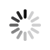#### Welcome!

×Forgot password?
Go back to Challenges list

Challenge

## Building a Basic Calculator

In this challenge you need to build a basic calculator that can perform actions like add, subtract, multiply and divide.The operation symbols are +, -, * and /.

###### Input Format
Each test case contains the first number, the symbol and the second number for the mathematical operation. All of them are separated by a blank space and all numbers are integers.

###### Output Format
For each test case output the operation result.

###### Sample Inputs
```15 + 2 -4 / 2```

###### Sample Outputs
```17 -2 ```

`512M`